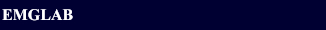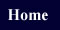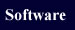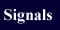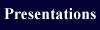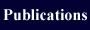EMGLAB FORUMEMGLAB FORUM >Comparing EMGLAB with Spike2

Subject:   Comparing EMGLAB with Spike2

 From:   Mike Pascoe Date:   18 Jul 2007 1:46 pm Reply via e-mail to Mike Pascoe.
Hello,

I am wanting to compare the output from EMGLAB decomposition with the template matching features of Spike2. Is there an established method of doing this as of now? I just want to present my findings in a common language to facilitate better comparison.

Let me know if there is an established technique, thanks,

-Mike
>> Reply to this message

Subject:   Re: Comparing EMGLAB with Spike2

 From:   Kevin McGill Date:   20 Jul 2007 4:40 pm Reply via e-mail to Kevin McGill.
Hi Mike,

Thanks for your message. As I understand, you intend to process the same signal with both EMGlab and Spike2 and are asking if there are established ways to compare the resulting MUAP trains.

Farina et al.  have a paper on this, but two caveats should be mentioned. First, they assume that one set of trains is correct, and they measure the accuracy of the other trains with respect to this set. In your situation you can't really say that one set is correct. Second, the formula they use for accuracy includes "true negatives," which leads to considerable over-inflation of the accuracy values. Carey and Clancy  calculate a confusion matrix that shows the correspondence between each unit in train 1 and each unit in train 2.

I would say that the proper way to compare two sets of trains, neither of which can be assumed to be correct, is to calculate the rate at which they agree. Suppose train 1a (train a in set 1) corresponds to train 2b. Then the rate of agreement for this train is given by:

Nmatch / (N1a + N2b - Nmatch)

where Nmatch is the number of discharges for which the two trains agree, N1a is the total number of discharges in train 1a, and N2b is the total number of discharges in train 2b.

If you have a lot of confidence in one of the methods, then the agreement rate will give you a sense of how well the other method did in comparison. If you don't have any more confidence in one that the other, the agreement rate will only tell you to what extent their results agreed. It can't tell you whether one did better than the other.

If you are analyzing multi-unit signals, then you can compute the agreement rate for each separate unit. One potential difficulty is that a particular unit might be present in one set but not the other, giving an agreement rate of 0. You'll have to decide then whether it makes sense to keep or exclude these cases if you average across units.

The EMGlab program does not yet have the ability to compare two different annotation files, but there are some auxiliary programs in the package for doing this.

readers/eaf_view.m is a stand-alone program that allows you to compare two annotation files. It reads files in either .eaf format or .ann format (the newer and older EMGlab formats). It can't read spike2 format, but perhaps you can convert your spike2 trains into a .ann file, which is just a text file with two entries on each line: a firing time (in seconds), and a unit number. The program matches the units in the two files and plots them in such a way as to highlight the discrepancies.

Here is a simple Matlab program that computes the agreement rates for two annotation files. It uses readers/eaf_compare,m, which computes and prints the confusion matrix.

[f,p] = uigetfile ('*', 'load first annotation file');
A1 = eaf_load (fullfile(p,f));
[f,p] = uigetfile ('*', 'load second annotation file');
A2 = eaf_load (fullfile(p,f));
s = eaf_compare (A1, A2, 'Print', 'on');
M = s.Confuse;
fprintf ('Agree ');
for i=1:length(s.MUmap);
j=s.MUmap(i);
if isnan(j);
fprintf ('%6.1f', 0);
else
agree_rate(i)=M(j,i)/(sum(M(j,:))+sum(M(:,i))-M(j,i));
fprintf ('%6.1f', agree_rate(i)*100);
end;
end;
fprintf ('
');

Let me know if you have any questions. I would be very interested to hear the results of your comparison.

Sincerely,
Kevin McGill

 Farina D, Colombo R, Merletti R, Olsen HB. Evaluation of intra-muscular EMG signal decomposition algorithms. J Electromyogr Kinesiol. 2001 Jun;11(3):175-87.

 Carey RM, Clancy EA. EMG decomposition annotation comparison method. Proc IEEE 31st Ann Northeast Bioeng Conf, 100-101, 2005. (Available at http://emglab.stanford.edu/emglab/Publications/publications.html).

Mike Pascoe wrote:
>Hello,
>
>I am wanting to compare the output from EMGLAB decomposition with the template matching features of Spike2. Is there an established method of doing this as of now? I just want to present my findings in a common language to facilitate better comparison.
>
>Let me know if there is an established technique, thanks,
>
>-Mike
>> Reply to this message